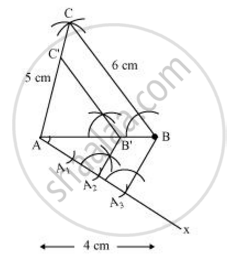# Construct a triangle of sides 4 cm, 5cm and 6cm and then a triangle similar to it whose sides are 2/3 of the corresponding sides of the first triangle. Give the justification of the construction. - Mathematics

Construct a triangle of sides 4 cm, 5cm and 6cm and then a triangle similar to it whose sides are 2/3 of the corresponding sides of the first triangle. Give the justification of the construction.

Construct a triangle with sides 5 cm, 4 cm and 6 cm. Then construct another triangle whose sides are 2/3 times the corresponding sides of first triangle.

#### Solution

Step 1

Draw a line segment AB = 4 cm. Taking point A as centre, draw an arc of 5 cm radius. Similarly, taking point B as its centre, draw an arc of 6 cm radius. These arcs will intersect each other at point C. Now, AC = 5 cm and BC = 6 cm and ΔABC is the required triangle.

Step 2

Draw a ray AX making an acute angle with line AB on the opposite side of vertex C.

Step 3

Locate 3 points A1, A2, A3 (as 3 is greater between 2 and 3) on line AX such that AA= A1A2 = A2A3.

Step 4

Join BA3 and draw a line through Aparallel to BA3 to intersect AB at point B'.

Step 5

Draw a line through B' parallel to the line BC to intersect AC at C'.

ΔAB'C' is the required triangle.Justification

The construction can be justified by proving that

AB' = 2/3AB, B'C'  = 2/3BC, AC' = 2/3 AC

By construction, we have B’C’ || BC

∴ ∠AB'C'= ∠ABC (Corresponding angles)

In ΔAB'C' and ΔABC,

∠AB'C' = ∠ABC (Proved above)

∠B'AC' = ∠BAC (Proved above)

∴ ΔAB'C' ~ ΔABC (AA similarity criterion)

=> (AB')/(AB) = (B'C')/(BC) = (AC')/(AC) ....(1)

In ΔAA2B' and ΔAA3B,

∠A2AB' = ∠A3AB (Common)

∠AA2B' = ∠AA3B (Corresponding angles)

∴ ΔAA2B' ∼ ΔAA3B (AA similarity criterion)

=> (AB')/(AB) = (

=> (AB')/(AB) = 2/3    ....(2)

From equations (1) and (2), we obtain

(AB')/(AB) = (B'C')/(BC) = (AC')/(AC) = 2/3

=>AB' = 2/3(AB), B'C' = 2/3(BC), AC' = 2/3(AC)

This justifies the construction.

Concept: Division of a Line Segment
Is there an error in this question or solution?
Chapter 11: Constructions - Exercise 11.1 [Page 220]

#### APPEARS IN

NCERT Class 10 Maths
Chapter 11 Constructions
Exercise 11.1 | Q 2 | Page 220
RD Sharma Class 10 Maths
Chapter 9 Constructions
Exercise 9.2 | Q 1 | Page 9

Share